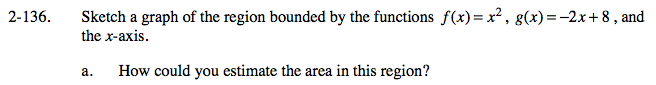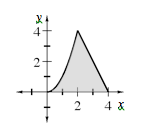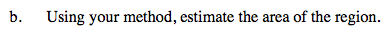### Home > CALC > Chapter 2 > Lesson 2.4.1 > Problem2-136

2-136.Graph the functions. Shade the region. Find all relevant intersections: Where does f(x) intersect with g(x)? Where does each function cross the x-axis? How many integrals will you need?

The functions intersect at (2, 4). The area should be approximated with rectangles or trapezoids for 0 ≤ x ≤ 2 and added to the area of the triangle for 2 ≤ x ≤ 4.$\text{Estimates will vary. area }\approx \frac{1}{500}\sum_{n=0}^{999}\Big(\frac{n}{500}\Big)^2+\frac{1}{2}(2)(4)\approx 6.66\text{ units}^2$